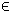## 4QP Problem

The general formulation in TOMLAB for a quadratic programming problem is:

 min x
f(x) =
1
2
xT F x + cT x

s/t
 xL ≤ x ≤ xU bL ≤ A x ≤ bU
(4)
where c, x, xL, xURn, FRn × n, ARm1 × n, and bL,bURm1. Equality constraints are defined by setting the lower bound equal to the upper bound, i.e. for constraint i: bL(i) = bU(i). Fixed variables are handled the same way.

Example problem:

 min x
f(x)=4x12+1x1x2+4x22+3x1−4x2

s/t
 x1+x2 ≤ 5 x1−x2 = 0 x1 ≥ 0 x2 ≥ 0
(5)

The following file defines this problem in TOMLAB.

File: tomlab/quickguide/qpQG.m

Open the file for viewing, and execute qpQG in Matlab.
```% qpQG is a small example problem for defining and solving
% quadratic programming problems using the TOMLAB format.

Name  = 'QP Example';
F     = [ 8   1        % Matrix F in 1/2 * x' * F * x + c' * x
1   8 ];
c     = [ 3  -4 ]';    % Vector c in 1/2 * x' * F * x + c' * x
A     = [ 1   1        % Constraint matrix
1  -1 ];
b_L   = [-inf  0  ]';  % Lower bounds on the linear constraints
b_U   = [  5   0  ]';  % Upper bounds on the linear constraints
x_L   = [  0   0  ]';  % Lower bounds on the variables
x_U   = [ inf inf ]';  % Upper bounds on the variables
x_0   = [  0   1  ]';  % Starting point
x_min = [-1 -1 ];      % Plot region lower bound parameters
x_max = [ 6  6 ];      % Plot region upper bound parameters

% Assign routine for defining a QP problem.
Prob = qpAssign(F, c, A, b_L, b_U, x_L, x_U, x_0, Name,...
[], [], [], x_min, x_max);

% Calling driver routine tomRun to run the solver.
% The 1 sets the print level after optimization.

Result = tomRun('qpSolve', Prob, 1);
%Result = tomRun('snopt', Prob, 1);
%Result = tomRun('sqopt', Prob, 1);
%Result = tomRun('cplex', Prob, 1);
%Result = tomRun('knitro', Prob, 1);
%Result = tomRun('conopt', Prob, 1);```